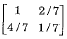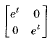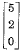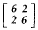# if A be an invertible matrix and inverse of 7A is , then matrix A is

1.

2.

3.

4.

4Explanation :
No Explanation available for this question

# solution(s) to the equations 2x+3y=1 x-y=4 4x-y=a Will exist if a equal to

1.  -33

2.  0

3.  9

4.  12

4

12

Explanation :
No Explanation available for this question

# matrix B=AT,where A is any matrix is

1.  Skew symmetric

2.  Symmetric about the second diagonal

3.  Always symmetric

4.  Another general matrix

4

Always symmetric

Explanation :
No Explanation available for this question

# if AT=A-1, where A is a real matrix, then A is

1.  Noemal

2.  Symmetric

3.  Hermitian

4.  Orthogonal

4

Orthogonal

Explanation :
No Explanation available for this question

# If A and B are non-zero squarematrices, then AB=I implies

1.  A and B are orthogonal

2.  A and B are singular

3.  B is singular

4.  A is singular

4

A and B are orthogonal

Explanation :
No Explanation available for this question

# eigen values of a square symmetric matrix are always

1.  Positive

2.  Real and imaginary

3.  Negative

4.  Real

4

Real

Explanation :
No Explanation available for this question

# I f A=, then state transition matrix eAt is

1.

2.

3.

4.

4Explanation :
No Explanation available for this question

# for matrix p= , if one of the eigen values is equal to -2, then which of the following is an eigen vector

1.

2.

3.

4.

4Explanation :
No Explanation available for this question

# if A= and A-1=, then (a+b) equals

1.  7/20

2.  3/20

3.  19/60

4.  11/20

4

7/20

Explanation :
No Explanation available for this question

# eigen values and corresponding eigen vectors of a 2x2 matrix are given by the matrix is Eigen value eigen vector λ 1=4 v1= λ 2=8 v2=

1.

2.

3.

4.

4